Courses

# Arithmetic and Logic Unit Computer Science Engineering (CSE) Notes | EduRev

## Electronics and Communication Engineering (ECE) : Arithmetic and Logic Unit Computer Science Engineering (CSE) Notes | EduRev

The document Arithmetic and Logic Unit Computer Science Engineering (CSE) Notes | EduRev is a part of the Electronics and Communication Engineering (ECE) Course Digital Electronics.
All you need of Electronics and Communication Engineering (ECE) at this link: Electronics and Communication Engineering (ECE)

Arithmetic and Logic Unit
ALU is the combinational circuit of that part of computer that actually performs arithmetic and logical operations on data. All of the other elements of computer system- control unit, registers, memory, I/O are their mainly to bring data into the ALU for it to process and then to take the result back out. An ALU & indeed all electronic components in computer are based on the use of simple digital logic device that can store binary digit and perform simple Boolean logic function. Figure indicates in general in general term how ALU is interconnected with rest of the processor.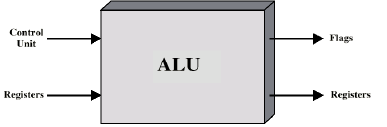Data are presented to ALU in register and the result of operation is stored in register. These registers are temporarily storage location within the processor that are connected by signal path to the ALU. The ALU may also set flags as the result of an operation. The flags values are also stored in registers within the processor. The control unit provides signals that control the operation of ALU and the movement of data into an out of ALU.

The design of ALU has three stages.

1. Design the arithmetic section
The basic component of arithmetic circuit is a parallel adder which is constructed with a number of full adder circuits connected in cascade. By controlling the data inputs to the parallel adder, it is possible to obtain different types of arithmetic operations. Below figure shows the arithmetic circuit and its functional table.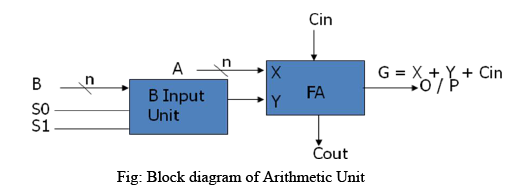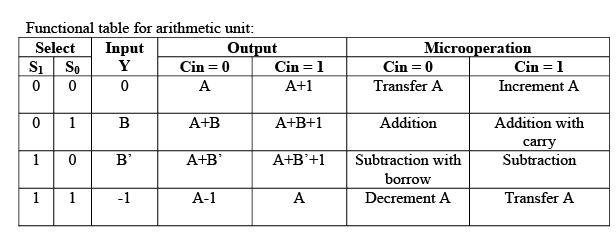2. Design the logical section
The basic components of logical circuit are AND, OR, XOR and NOT gate circuits connected accordingly. Below figure shows a circuit that generates four basic logic micro-operations. It consists of four gates and a multiplexer. Each of four logic operations is generated through a gate that performs the required logic. The two selection input S1 and S0 choose one of the data inputs of the multiplexer and directs its value to the output. Functional table lists the logic operations.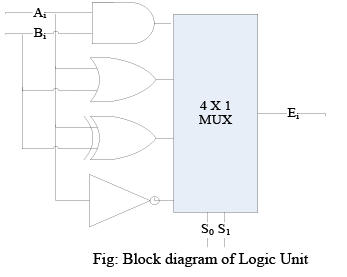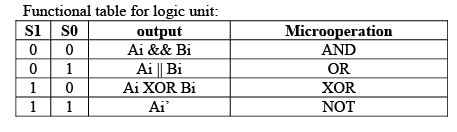3. Combine these 2 sections to form the ALU
Below figure shows a combined circuit of ALU where n data input from A are combined with n data input from B to generate the result of an operation at the G output line. ALU has a number of selection lines used to determine the operation to be performed. The selection lines are decoded with the ALU so that selection lines can specify distinct operations. The mode select S2 differentiate between arithmetic and logical operations. The two functions select S1 and S0 specify the particular arithmetic and logic operations to be performed. With three selection lines, it is possible to specify arithmetic operation with S2 at 0 and logical operation with S2 at 1.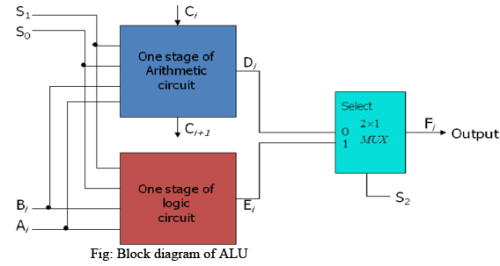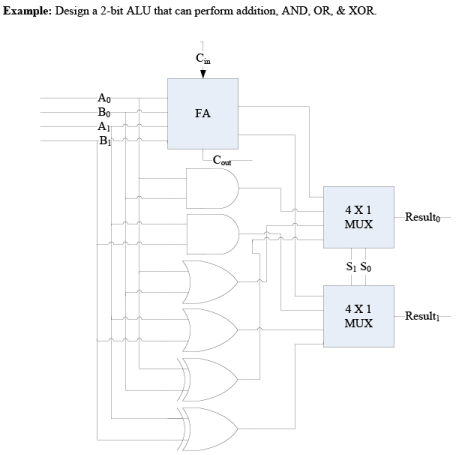Offer running on EduRev: Apply code STAYHOME200 to get INR 200 off on our premium plan EduRev Infinity!

## Digital Electronics

92 videos|91 docs|12 tests

,

,

,

,

,

,

,

,

,

,

,

,

,

,

,

,

,

,

,

,

,

;